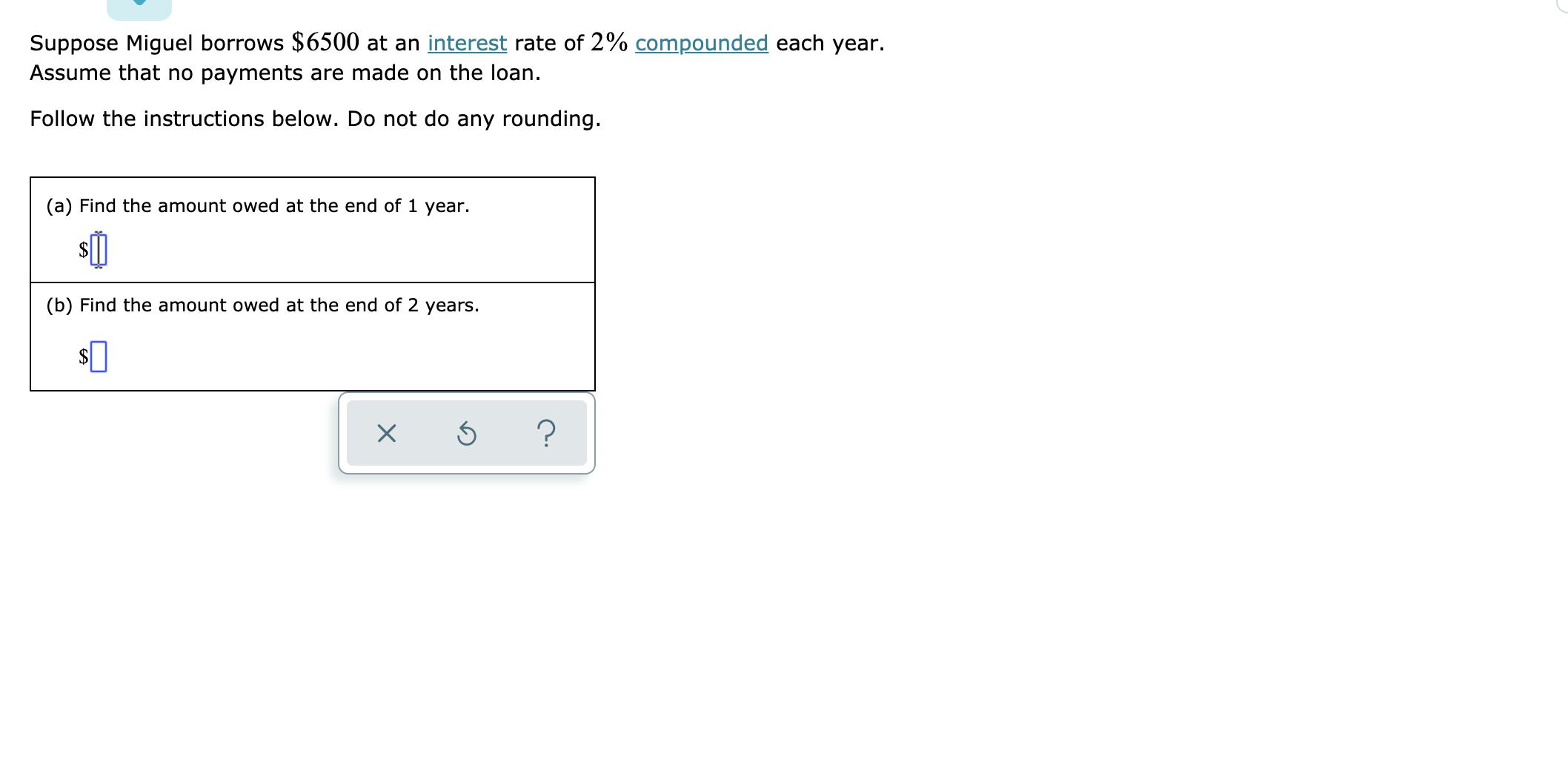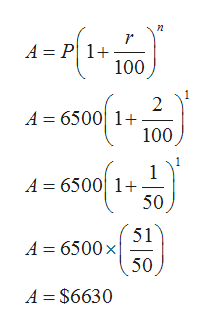Suppose Miguel borrows \$6500 at an interest rate of 2% compounded each year.Assume that no payments are made on the loan.Follow the instructions below. Do not do any rounding.(a) Find the amount owed at the end of 1 year.(b) Find the amount owed at the end of 2 years

Question

See attachedhelp_outlineImage TranscriptioncloseSuppose Miguel borrows \$6500 at an interest rate of 2% compounded each year. Assume that no payments are made on the loan. Follow the instructions below. Do not do any rounding. (a) Find the amount owed at the end of 1 year. (b) Find the amount owed at the end of 2 years fullscreen
Step 1

Given P=\$6500 and r=2% compounded annually. So for finding the final amount we use the formula where A=final value, P= principal value, r= annual rate, n= number of year

Step 2

(a)For n=...help_outlineImage Transcriptioncloser A P 1 100 2 A 6500 1 100 1 A 6500 1+ 50 51 A 6500x 50 A \$6630 fullscreen

Want to see the full answer?

See Solution

Want to see this answer and more?

Our solutions are written by experts, many with advanced degrees, and available 24/7

See Solution
Tagged in

Other